# Fixed points in quantum gravity and cosmology quantum

• Slides: 77Fixed points in quantum gravity and cosmologyquantum gravity with scalar field – the role of scale symmetry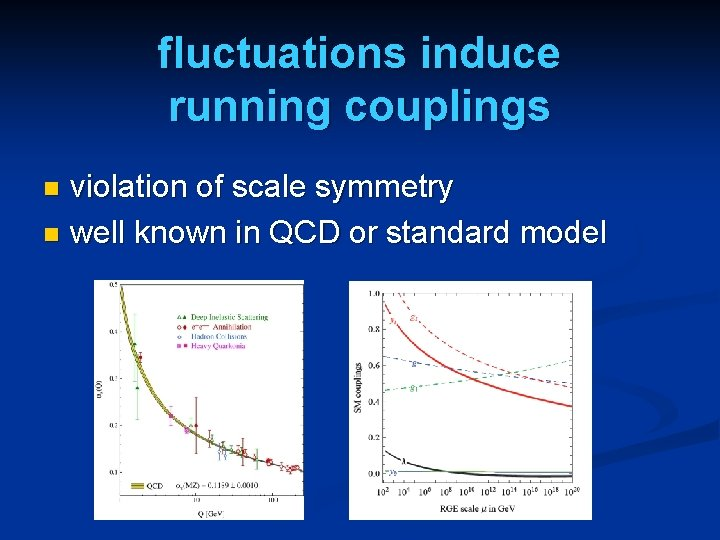fluctuations induce running couplings violation of scale symmetry n well known in QCD or standard model n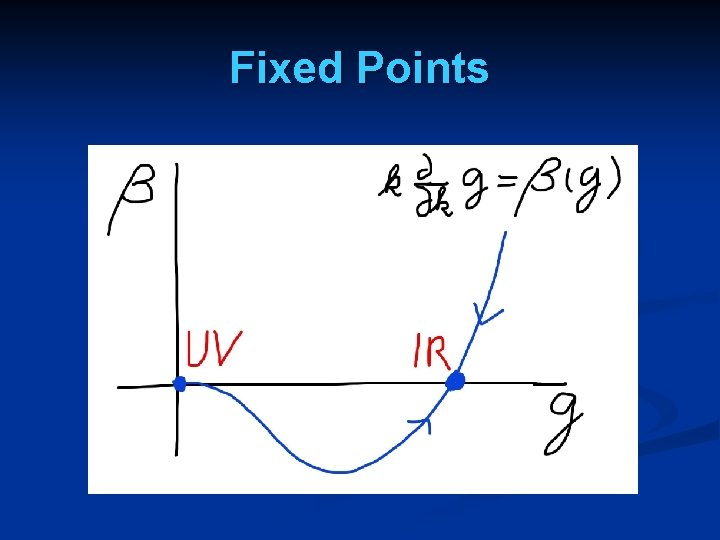Fixed Points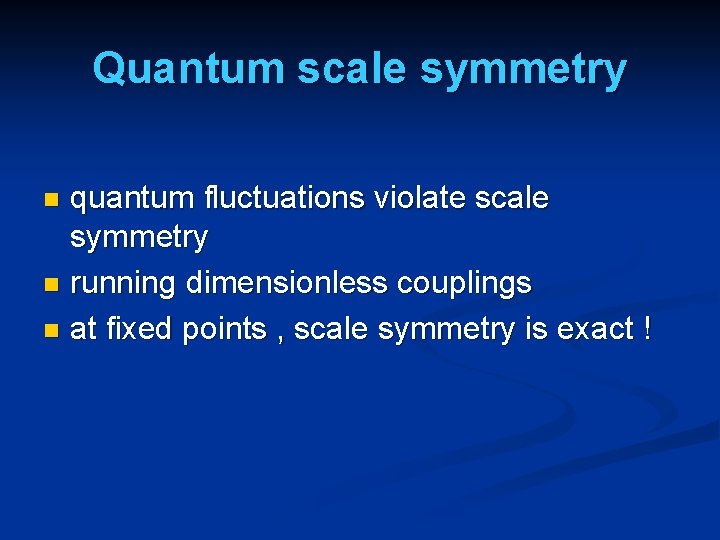Quantum scale symmetry quantum fluctuations violate scale symmetry n running dimensionless couplings n at fixed points , scale symmetry is exact ! nScale symmetry no scale symmetry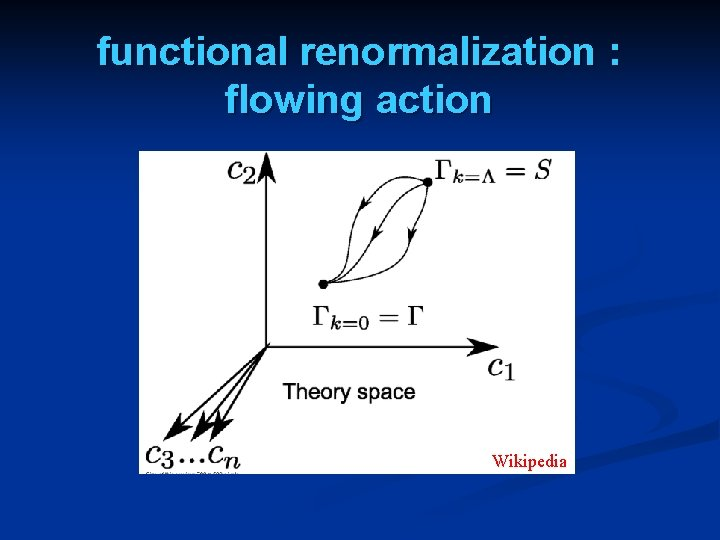functional renormalization : flowing action Wikipedia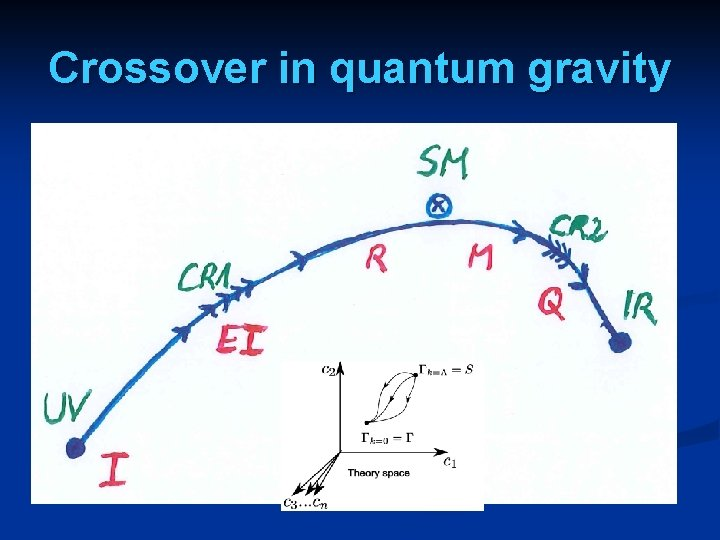Crossover in quantum gravity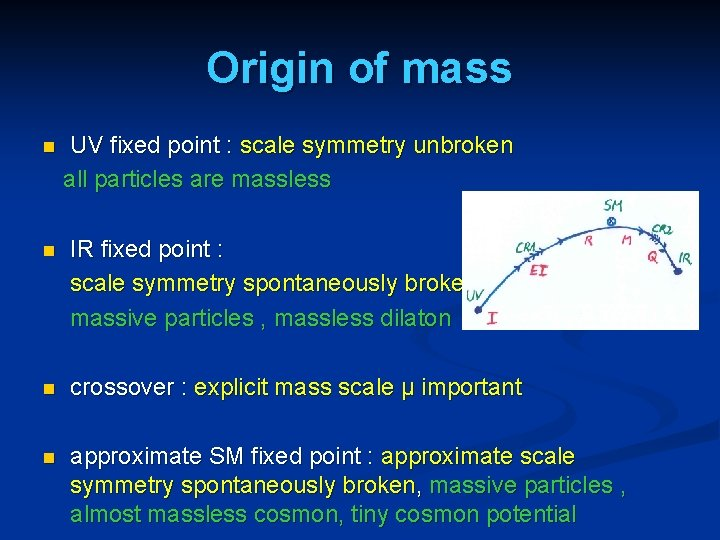Origin of mass n UV fixed point : scale symmetry unbroken all particles are massless n IR fixed point : scale symmetry spontaneously broken, massive particles , massless dilaton n crossover : explicit mass scale μ important n approximate SM fixed point : approximate scale symmetry spontaneously broken, massive particles , almost massless cosmon, tiny cosmon potential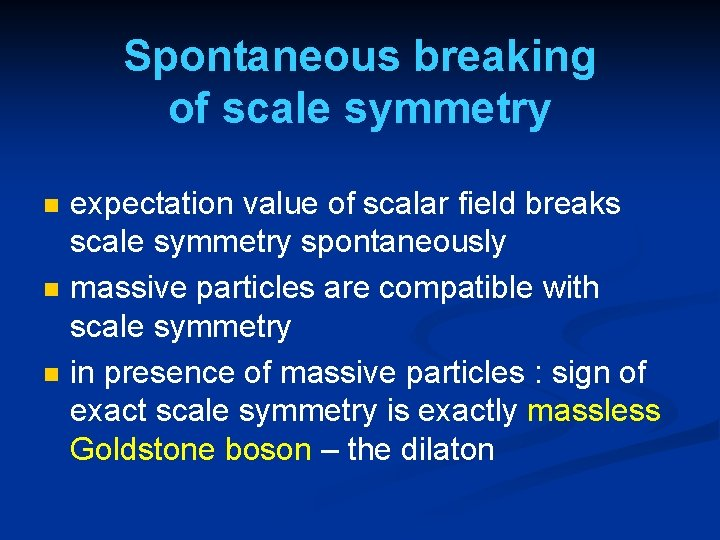Spontaneous breaking of scale symmetry n n n expectation value of scalar field breaks scale symmetry spontaneously massive particles are compatible with scale symmetry in presence of massive particles : sign of exact scale symmetry is exactly massless Goldstone boson – the dilaton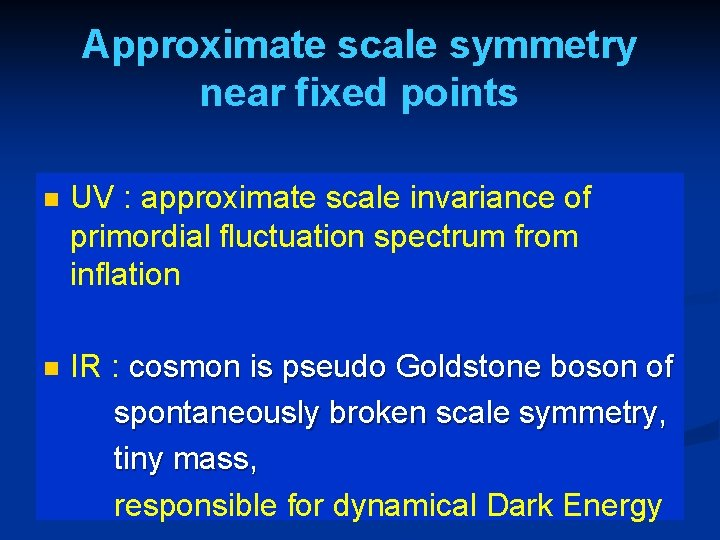Approximate scale symmetry near fixed points n UV : approximate scale invariance of primordial fluctuation spectrum from inflation n IR : cosmon is pseudo Goldstone boson of spontaneously broken scale symmetry, tiny mass, responsible for dynamical Dark EnergyAsymptotic safety if UV fixed point exists : quantum gravity is non-perturbatively renormalizable ! S. Weinberg , M. Reuter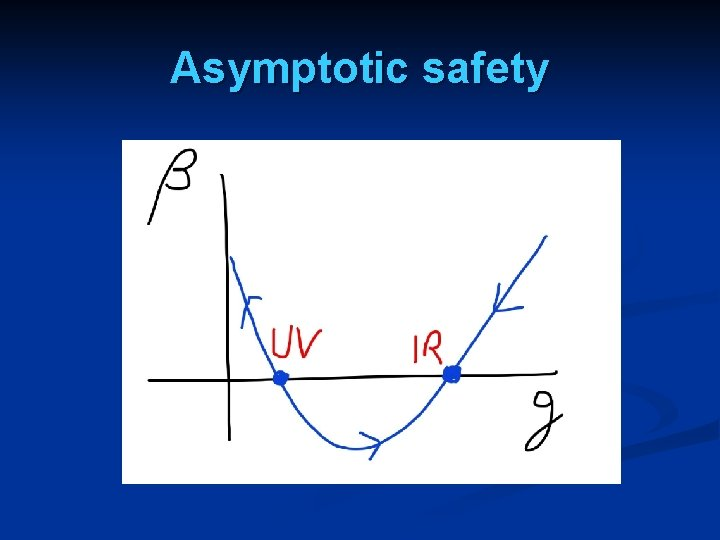Asymptotic safety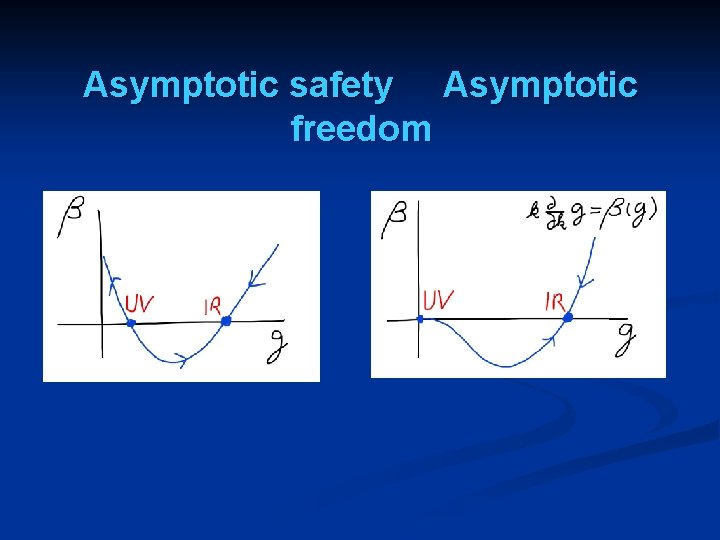Asymptotic safety Asymptotic freedoma prediction…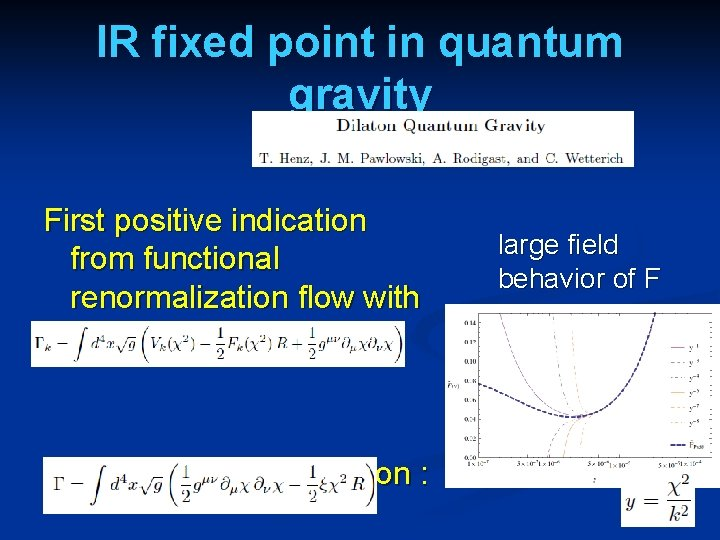IR fixed point in quantum gravity First positive indication from functional renormalization flow with truncation : fixed point effective action : large field behavior of FPossible consequences of crossover in quantum gravity Realistic model for inflation and dark energy with single scalar field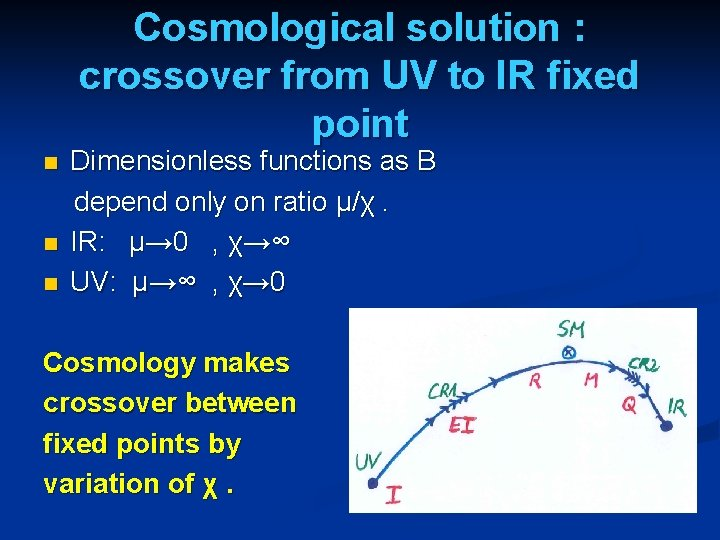Cosmological solution : crossover from UV to IR fixed point n n n Dimensionless functions as B depend only on ratio μ/χ. IR: μ→ 0 , χ→∞ UV: μ→∞ , χ→ 0 Cosmology makes crossover between fixed points by variation of χ.renormalization flow and cosmological evolution n renormalization flow as function of µ is mapped by dimensionless functions to n field dependence of effective action on scalar field χ translates by solution of field equation to n dependence of cosmology an time t or ηSimplicity simple description of all cosmological epochs natural incorporation of Dark Energy : n inflation n Early Dark Energy n present Dark Energy dominated epoch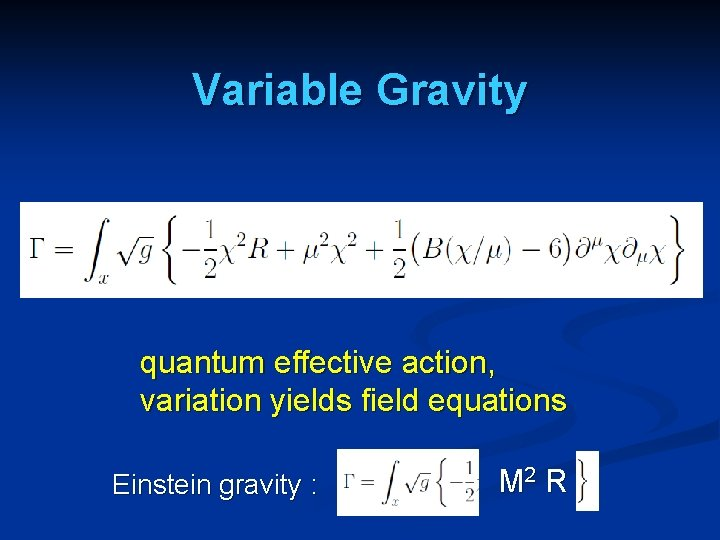Variable Gravity quantum effective action, variation yields field equations Einstein gravity : M 2 R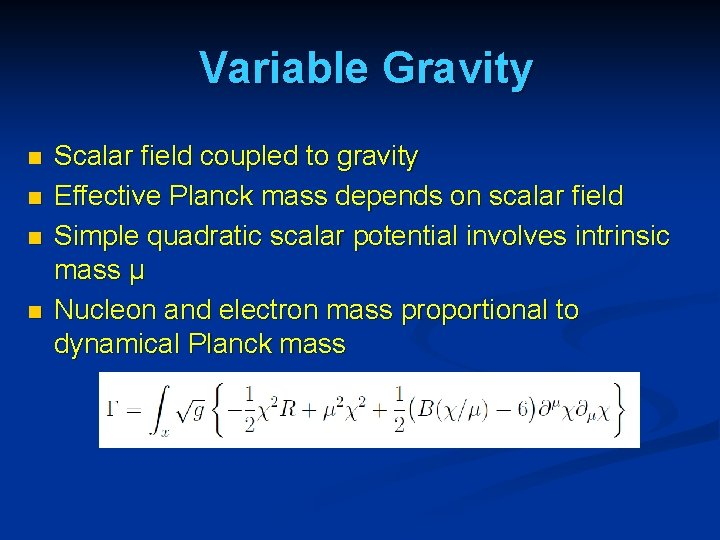Variable Gravity n n Scalar field coupled to gravity Effective Planck mass depends on scalar field Simple quadratic scalar potential involves intrinsic mass μ Nucleon and electron mass proportional to dynamical Planck mass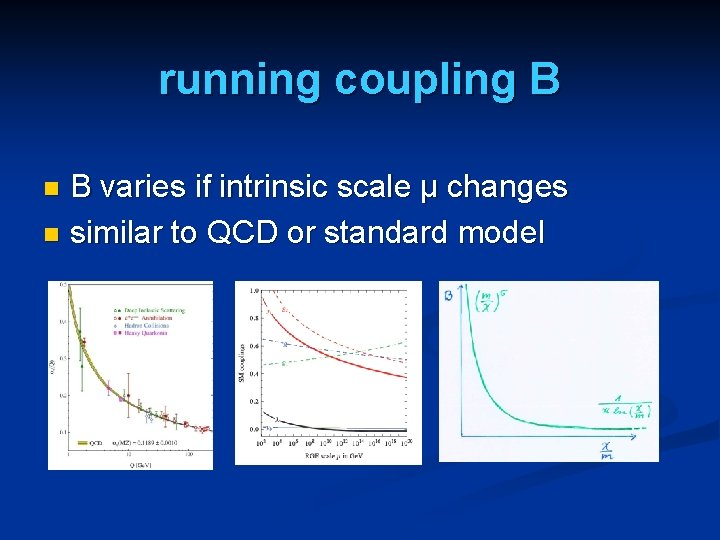running coupling B B varies if intrinsic scale µ changes n similar to QCD or standard model nKinetial B : Crossover between two fixed points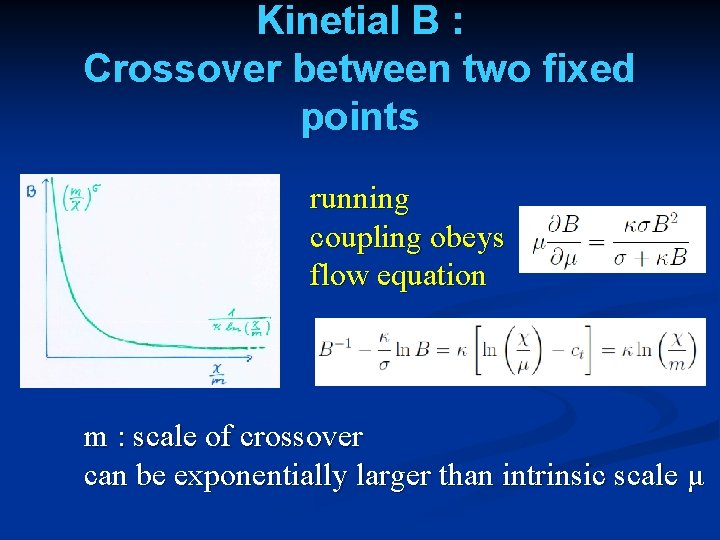Kinetial B : Crossover between two fixed points running coupling obeys flow equation m : scale of crossover can be exponentially larger than intrinsic scale μ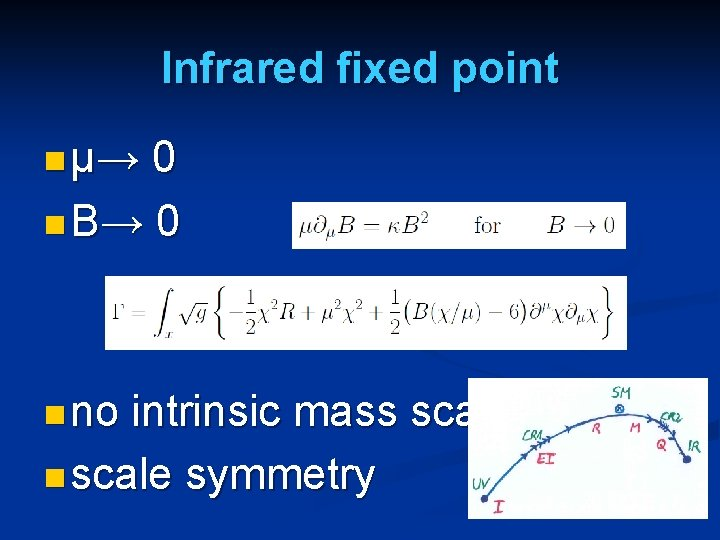Infrared fixed point n μ→ 0 n B→ 0 n no intrinsic mass scale n scale symmetry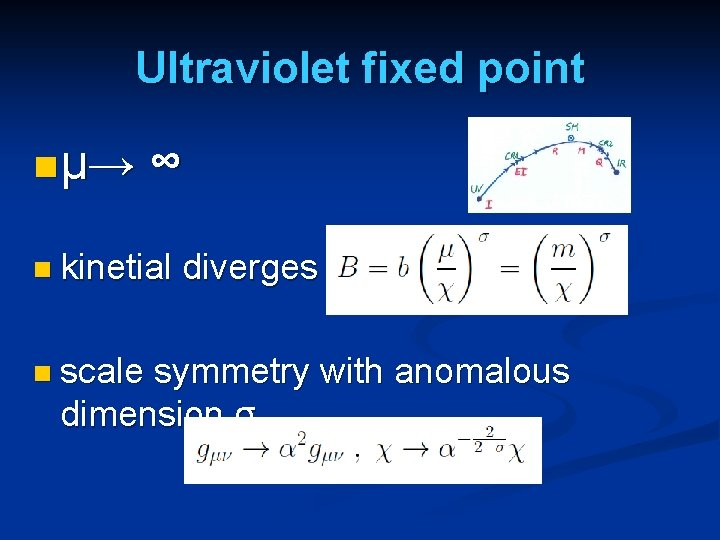Ultraviolet fixed point n μ→ ∞ n kinetial n scale diverges symmetry with anomalous dimension σ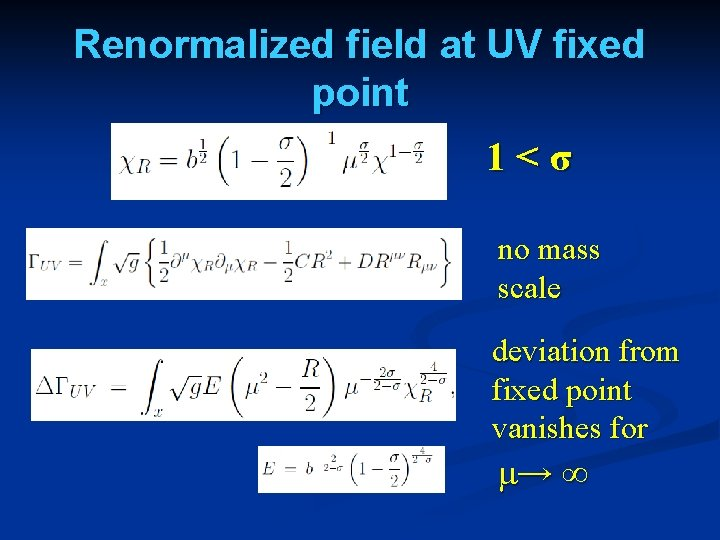Renormalized field at UV fixed point 1<σ no mass scale deviation from fixed point vanishes for μ→ ∞Cosmological solution : crossover from UV to IR fixed point n n n Dimensionless functions as B depend only on ratio μ/χ. IR: μ→ 0 , χ→∞ UV: μ→∞ , χ→ 0 Cosmology makes crossover between fixed points by variation of χ.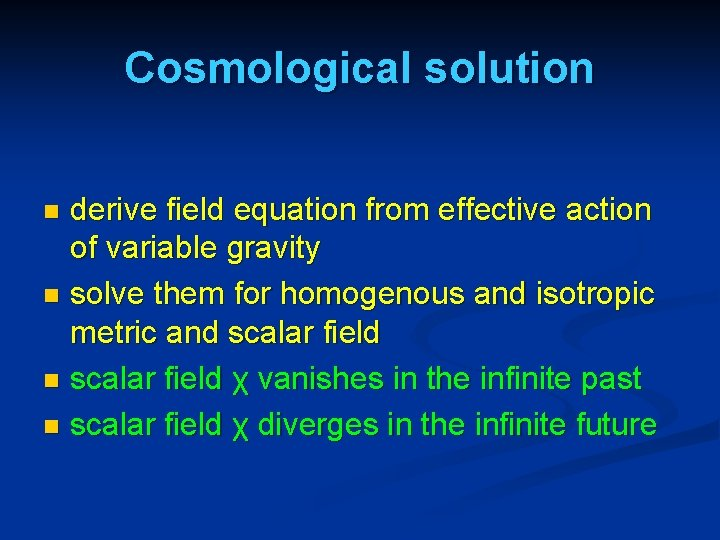Cosmological solution derive field equation from effective action of variable gravity n solve them for homogenous and isotropic metric and scalar field n scalar field χ vanishes in the infinite past n scalar field χ diverges in the infinite future nNo tiny dimensionless parameters ( except gauge hierarchy ) n n one mass scale μ = 2 10 -33 one time scale -1 μ = 10 e. V 10 yr Planck mass does not appear as parameter n Planck mass grows large dynamically n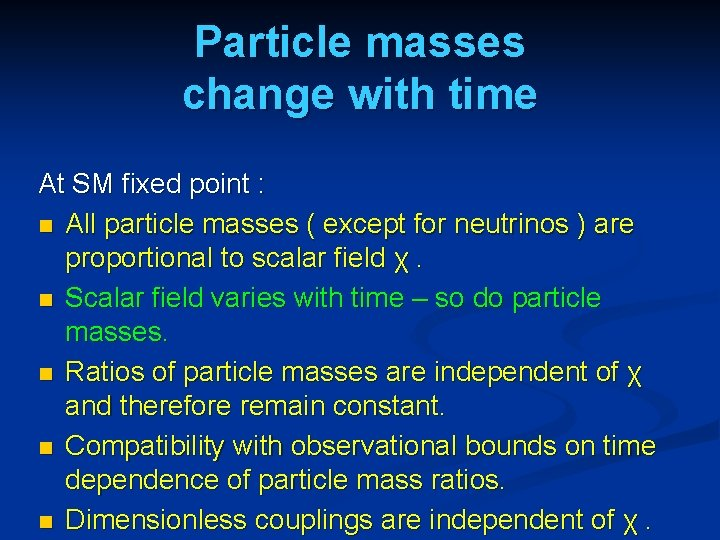Particle masses change with time At SM fixed point : n All particle masses ( except for neutrinos ) are proportional to scalar field χ. n Scalar field varies with time – so do particle masses. n Ratios of particle masses are independent of χ and therefore remain constant. n Compatibility with observational bounds on time dependence of particle mass ratios. n Dimensionless couplings are independent of χ.Four-parameter model n n n model has four dimensionless parameters three in kinetial B : σ ~ 2. 5 κ ~ 0. 5 ct ~ 14 ( or m/μ ) one parameter for present growth rate of neutrino mass over electron mass : γ ~ 8 + standard model particles and dark matter : sufficient for realistic cosmology from inflation to dark energy no more free parameters than ΛCDMCosmological solution n scalar field χ vanishes in the infinite past n scalar field χ diverges in the infinite future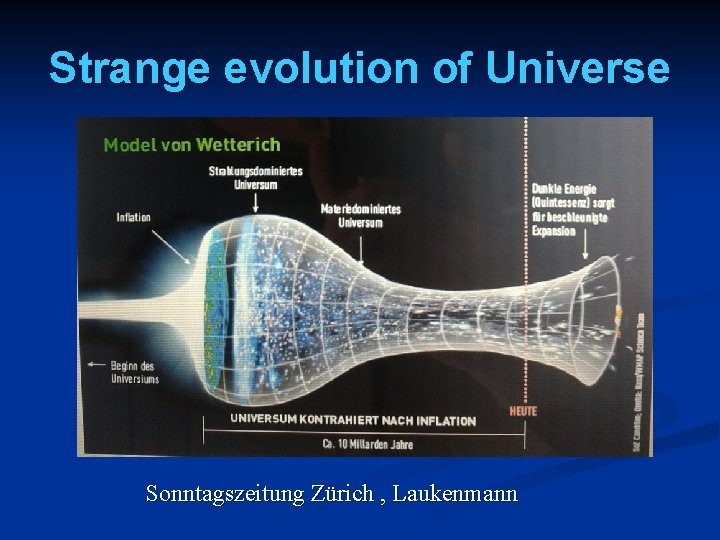Strange evolution of Universe Sonntagszeitung Zürich , Laukenmann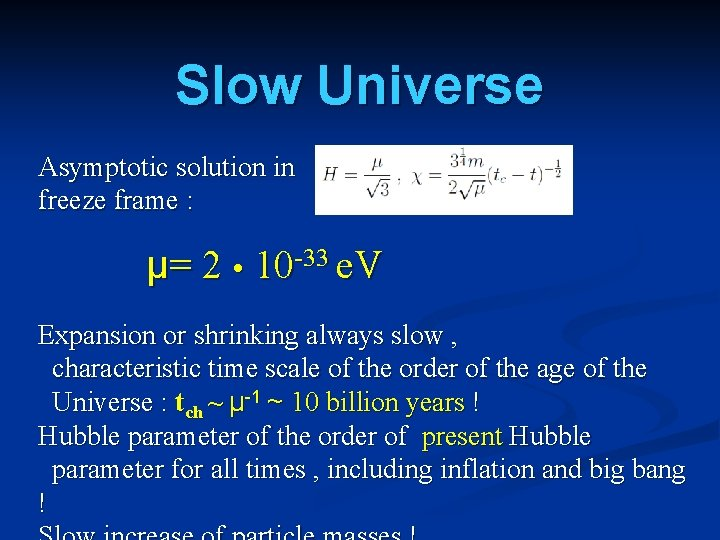Slow Universe Asymptotic solution in freeze frame : μ= 2 10 -33 e. V Expansion or shrinking always slow , characteristic time scale of the order of the age of the Universe : tch ~ μ-1 ~ 10 billion years ! Hubble parameter of the order of present Hubble parameter for all times , including inflation and big bang !Model is compatible with present observations Together with variation of neutrino mass over electron mass in present cosmological epoch : model is compatible with all present observations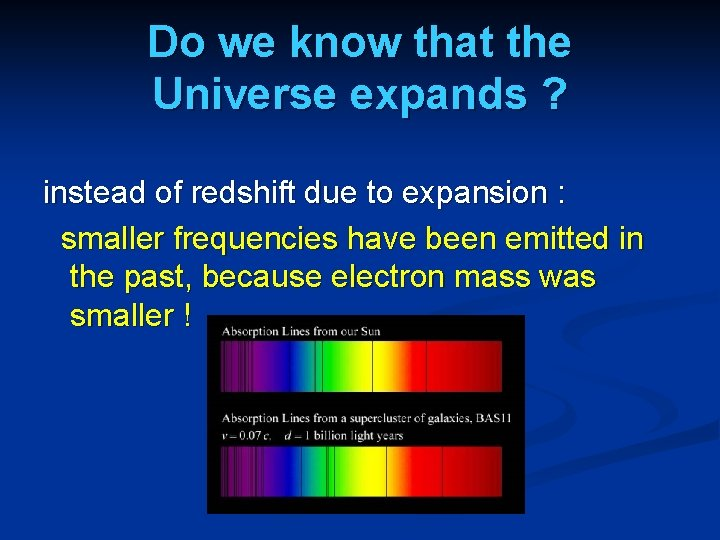Do we know that the Universe expands ? instead of redshift due to expansion : smaller frequencies have been emitted in the past, because electron mass was smaller !What is increasing ? Ratio of distance between galaxies over size of atoms ! atom size constant : expanding geometry alternative : shrinking size of atoms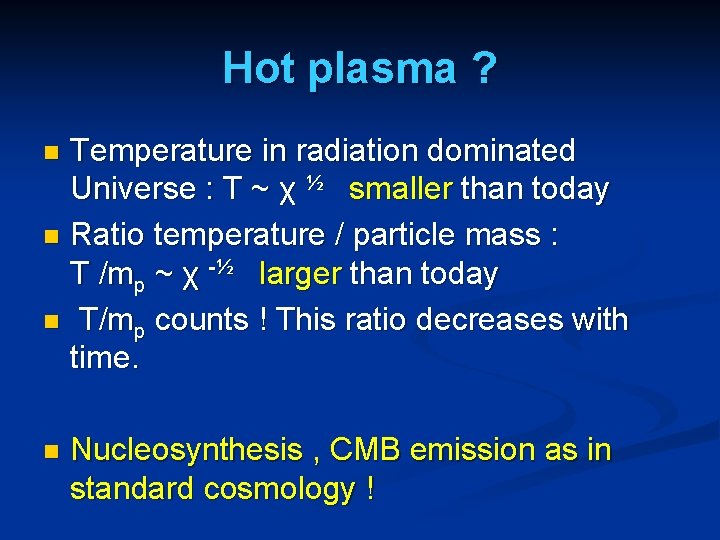Hot plasma ? Temperature in radiation dominated Universe : T ~ χ ½ smaller than today n Ratio temperature / particle mass : T /mp ~ χ -½ larger than today n T/mp counts ! This ratio decreases with time. n n Nucleosynthesis , CMB emission as in standard cosmology !Einstein frame “Weyl scaling” maps variable gravity model to Universe with fixed masses and standard expansion history. n Exact equivalence of different frames ! n n Standard gravity coupled to scalar field. n Only neutrino masses are growing.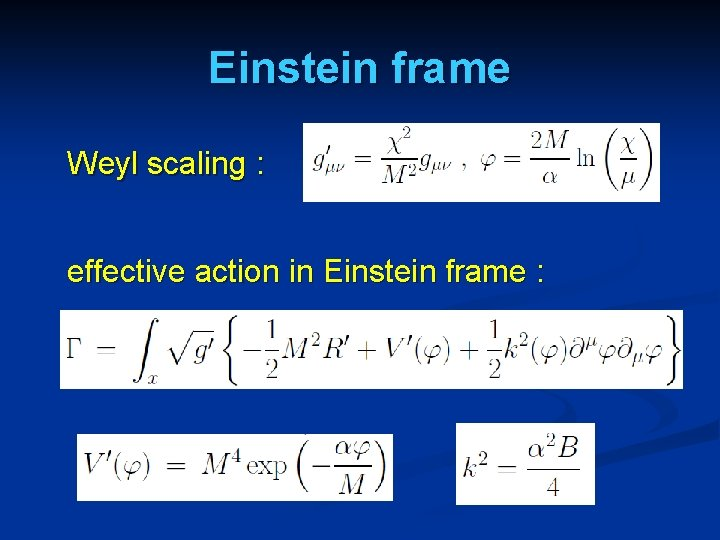Einstein frame Weyl scaling : effective action in Einstein frame :Field relativity : different pictures of cosmology same physical content can be described by different pictures n related by field – redefinitions , e. g. Weyl scaling , conformal scaling of metric n which picture is usefull ? n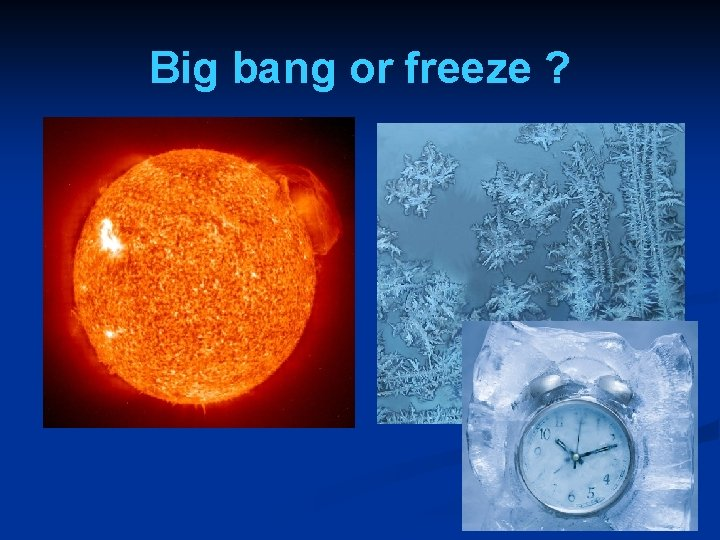Big bang or freeze ?Big bang or freeze ? just two ways of looking at same physics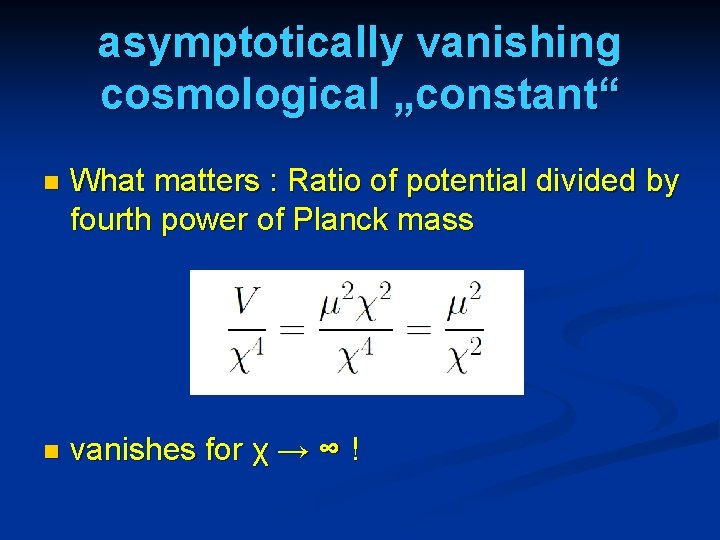asymptotically vanishing cosmological „constant“ n What matters : Ratio of potential divided by fourth power of Planck mass n vanishes for χ → ∞ !small dimensionless number ? needs two intrinsic mass scales n V and M ( cosmological constant and Planck mass ) n variable Planck mass moving to infinity , with fixed V: ratio vanishes asymptotically ! nEinstein frame Weyl scaling : effective action in Einstein frame :Infinite past : slow inflation σ = 2 : field equations solution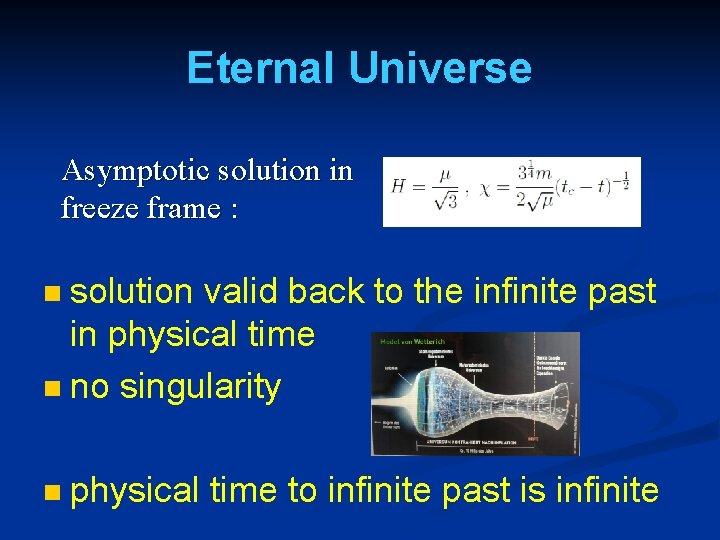Eternal Universe Asymptotic solution in freeze frame : solution valid back to the infinite past in physical time n no singularity n n physical time to infinite past is infinite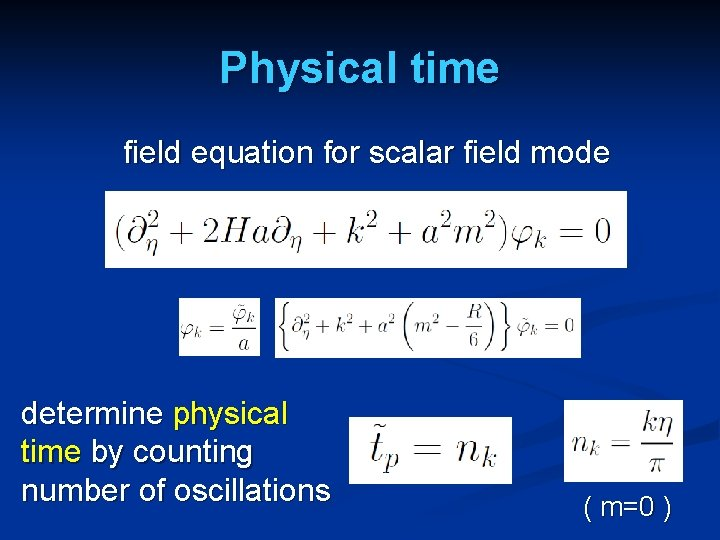Physical time field equation for scalar field mode determine physical time by counting number of oscillations ( m=0 )Big bang singularity in Einstein frame is field singularity ! choice of frame with constant particle masses is not well suited if physical masses go to zero !Inflation solution for small χ : inflationary epoch kinetial characterized by anomalous dimension σ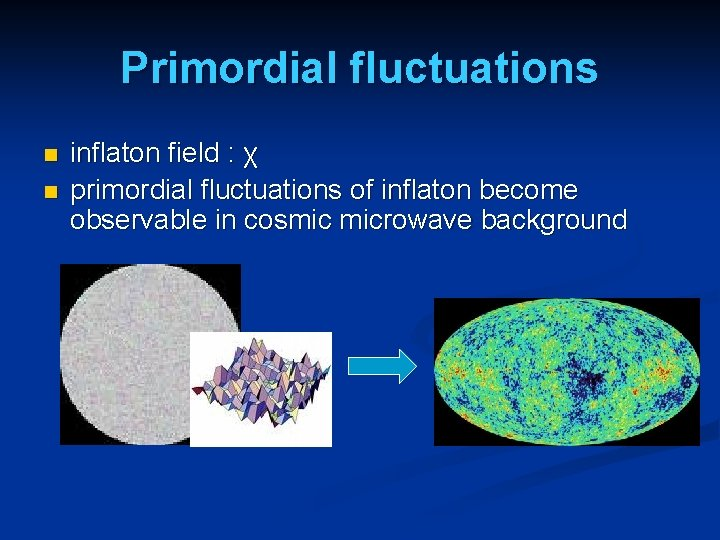Primordial fluctuations n n inflaton field : χ primordial fluctuations of inflaton become observable in cosmic microwave background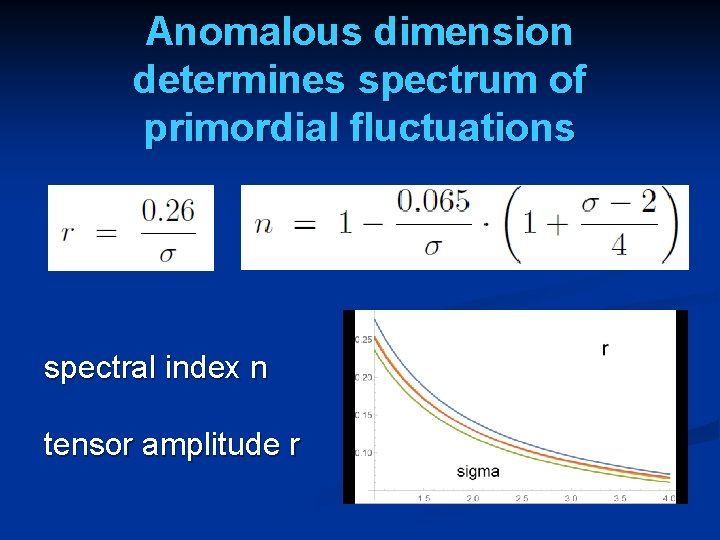Anomalous dimension determines spectrum of primordial fluctuations spectral index n tensor amplitude r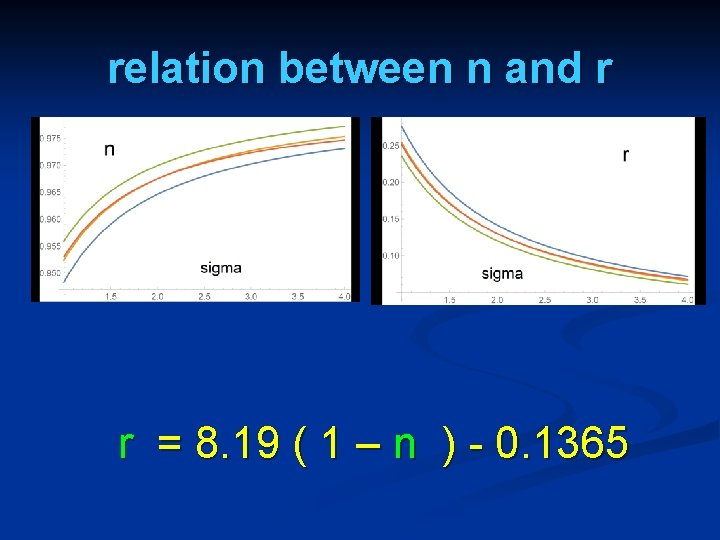relation between n and r r = 8. 19 ( 1 – n ) - 0. 1365Amplitude of density fluctuations small because of logarithmic running near UV fixed point ! σ=1 N : number of e – foldings at horizon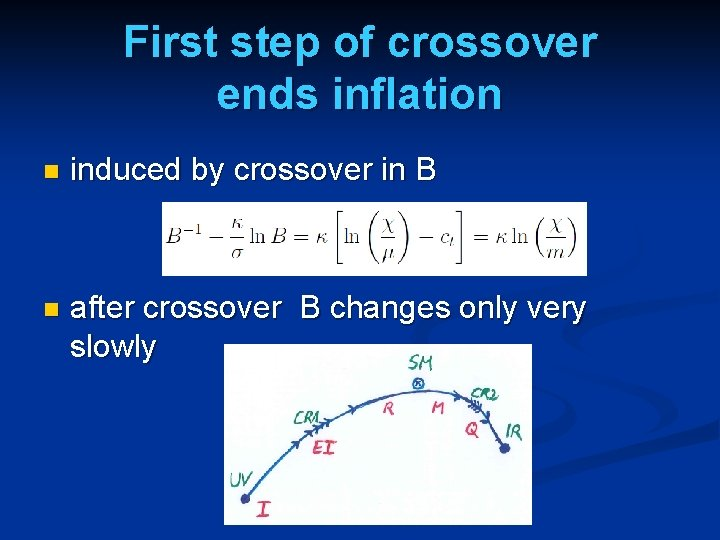First step of crossover ends inflation n induced by crossover in B n after crossover B changes only very slowly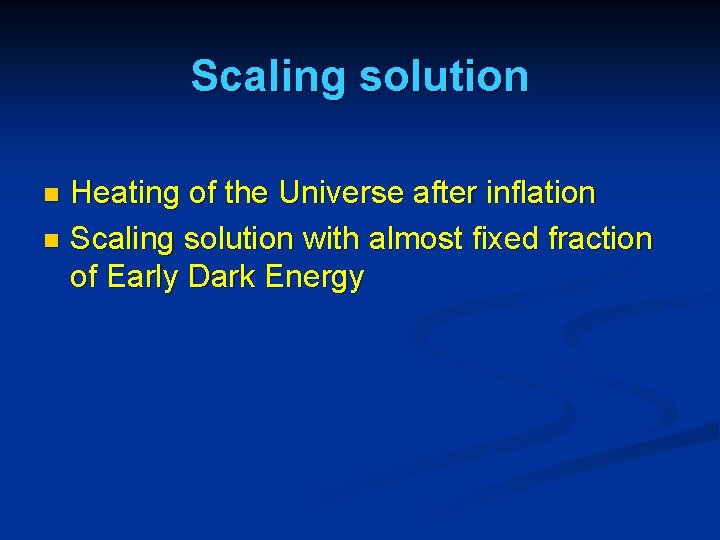Scaling solution Heating of the Universe after inflation n Scaling solution with almost fixed fraction of Early Dark Energy nCosmon inflation Unified picture of inflation and dynamical dark energy Cosmon and inflaton are the same scalar fieldQuintessence Dynamical dark energy , generated by scalar field (cosmon ) C. Wetterich, Nucl. Phys. B 302(1988)668, 24. 9. 87 P. J. E. Peebles, B. Ratra, Ap. J. Lett. 325(1988)L 17, 20. 10. 87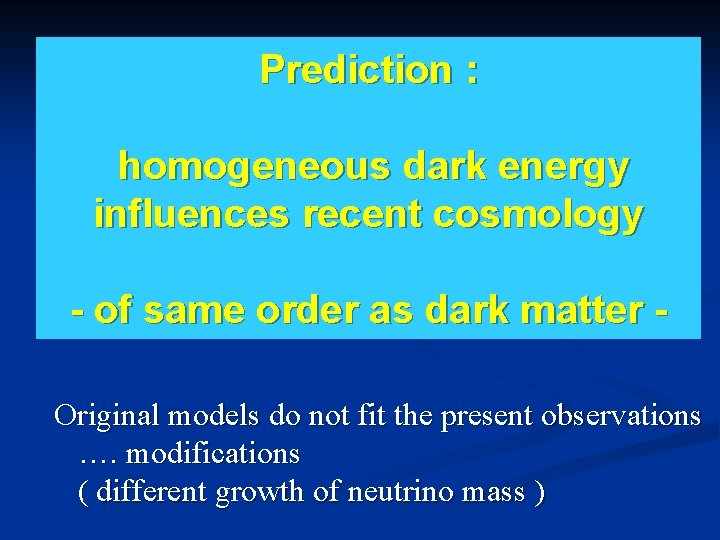Prediction : homogeneous dark energy influences recent cosmology - of same order as dark matter Original models do not fit the present observations …. modifications ( different growth of neutrino mass )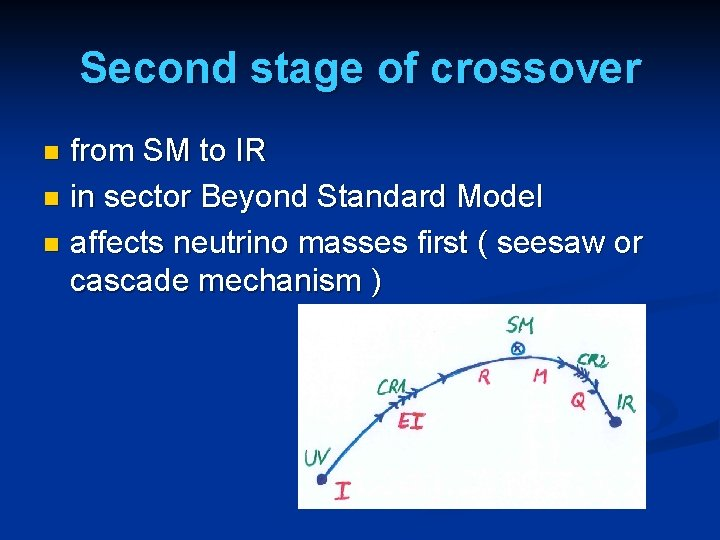Second stage of crossover from SM to IR n in sector Beyond Standard Model n affects neutrino masses first ( seesaw or cascade mechanism ) nVarying particle masses at onset of second crossover All particle masses except for neutrinos are proportional to χ. n Ratios of particle masses remain constant. n Compatibility with observational bounds on time dependence of particle mass ratios. n Neutrino masses show stronger increase with χ , such that ratio neutrino mass over electron mass grows. n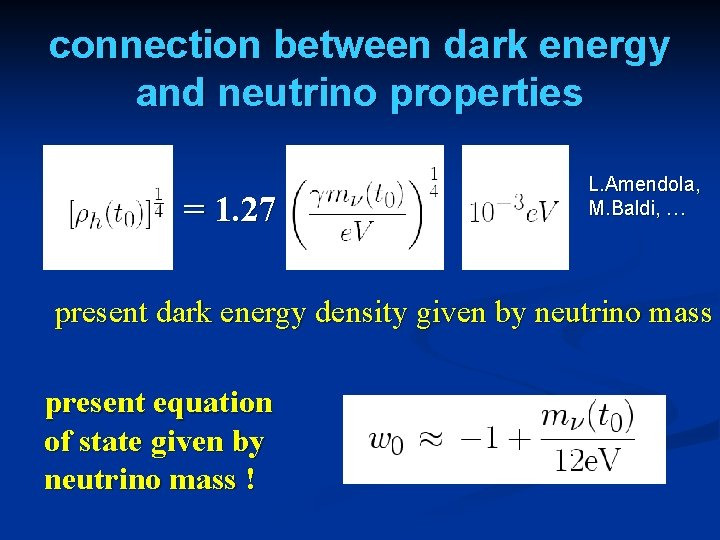connection between dark energy and neutrino properties = 1. 27 L. Amendola, M. Baldi, … present dark energy density given by neutrino mass present equation of state given by neutrino mass !Compatibility with observations and possible tests Realistic inflation model n Almost same prediction for radiation, matter, and Dark Energy domination as ΛCDM n Presence of small fraction of Early Dark Energy n Large neutrino lumps n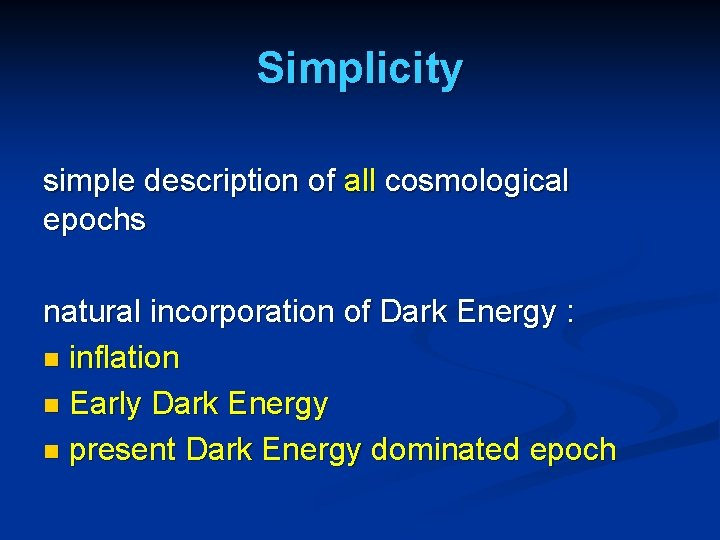Simplicity simple description of all cosmological epochs natural incorporation of Dark Energy : n inflation n Early Dark Energy n present Dark Energy dominated epoch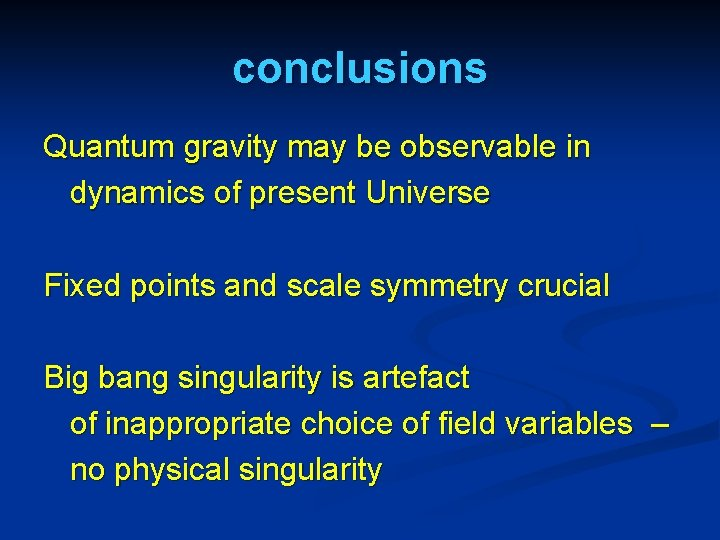conclusions Quantum gravity may be observable in dynamics of present Universe Fixed points and scale symmetry crucial Big bang singularity is artefact of inappropriate choice of field variables – no physical singularity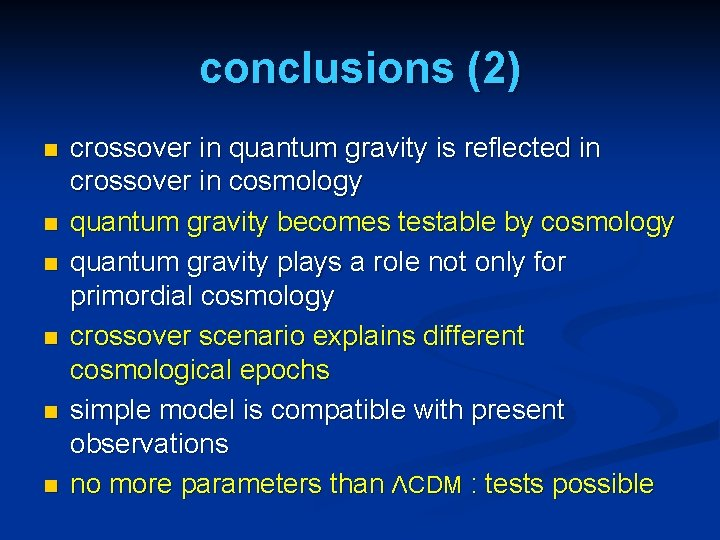conclusions (2) n n n crossover in quantum gravity is reflected in crossover in cosmology quantum gravity becomes testable by cosmology quantum gravity plays a role not only for primordial cosmology crossover scenario explains different cosmological epochs simple model is compatible with present observations no more parameters than ΛCDM : tests possible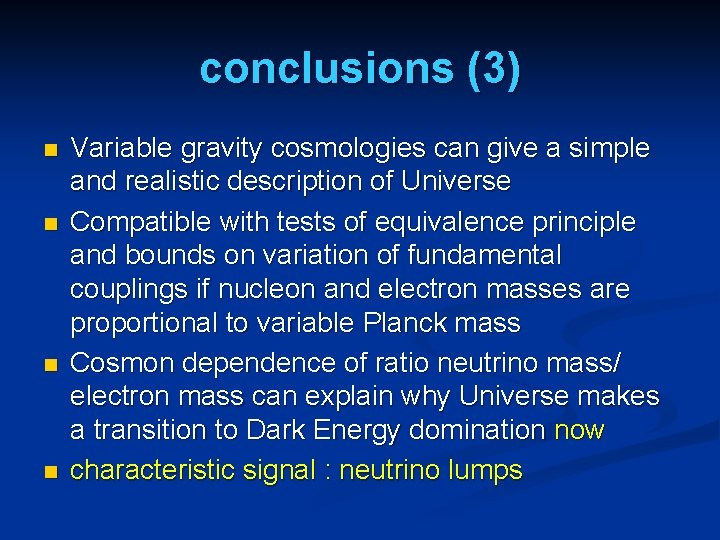conclusions (3) n n Variable gravity cosmologies can give a simple and realistic description of Universe Compatible with tests of equivalence principle and bounds on variation of fundamental couplings if nucleon and electron masses are proportional to variable Planck mass Cosmon dependence of ratio neutrino mass/ electron mass can explain why Universe makes a transition to Dark Energy domination now characteristic signal : neutrino lumpsend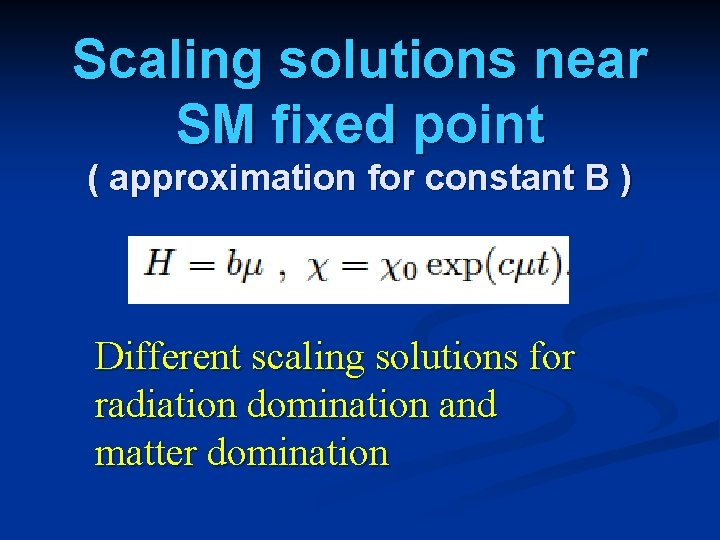Scaling solutions near SM fixed point ( approximation for constant B ) Different scaling solutions for radiation domination and matter domination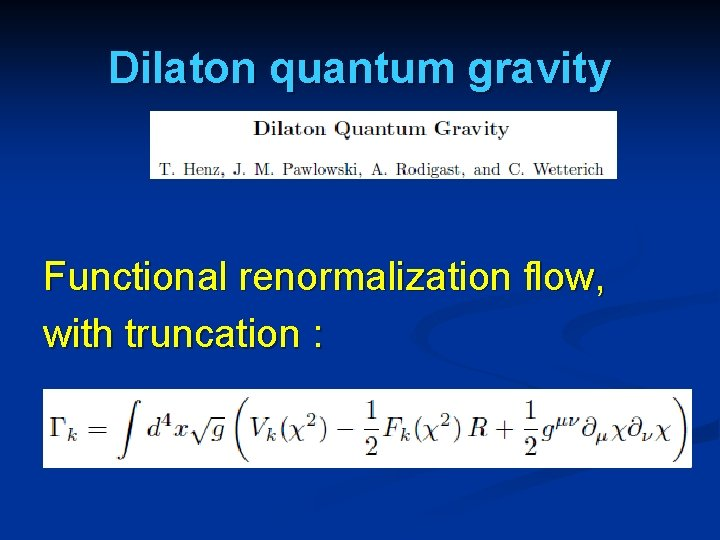Dilaton quantum gravity Functional renormalization flow, with truncation :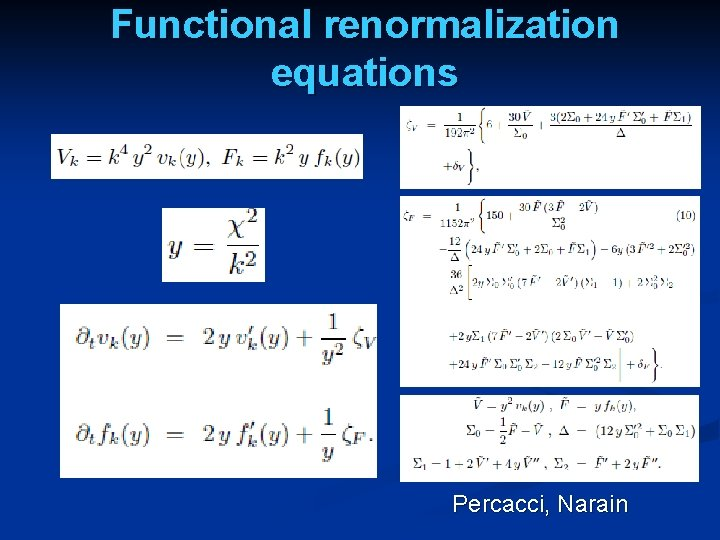Functional renormalization equations Percacci, Narain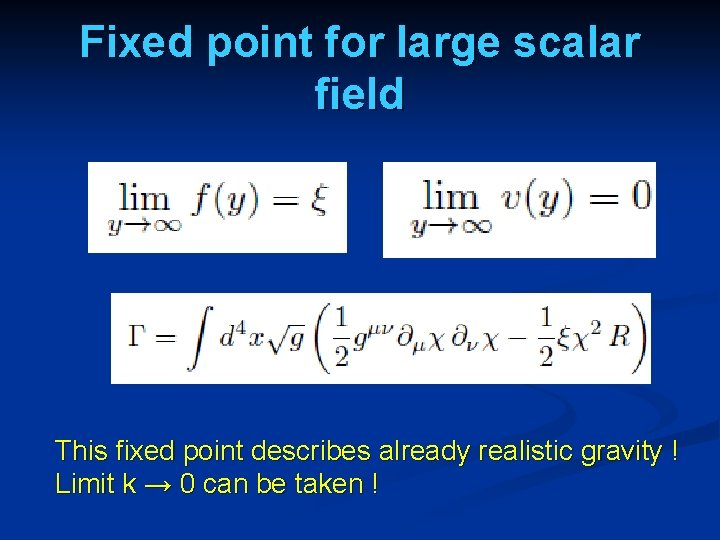Fixed point for large scalar field This fixed point describes already realistic gravity ! Limit k → 0 can be taken !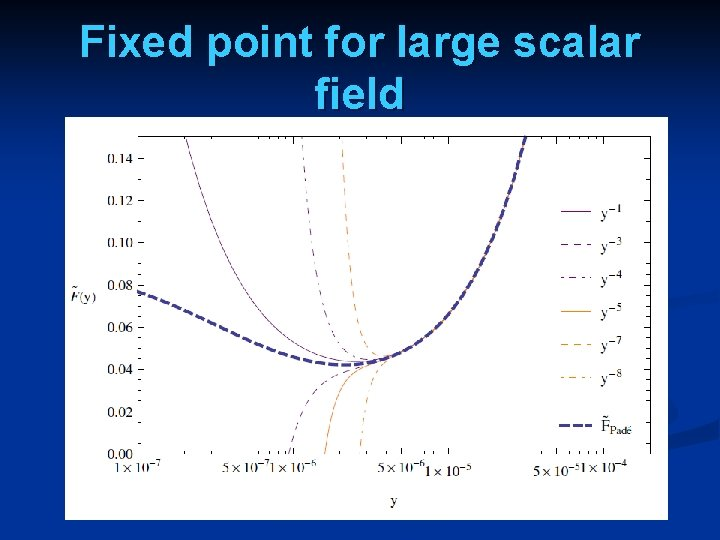Fixed point for large scalar fieldVicinity of fixed point solution : Cosmology with dynamical dark energy ! Cosmological constant vanishes asymptotically !